6401.0.60.005 - Information Paper: An Implementation Plan to Annually Re-weight the Australian CPI, 2017
Latest ISSUE Released at 11:30 AM (CANBERRA TIME) 19/06/2017  First Issue
 Page tools: .mffeedback,#pjs { display :none; } Enable Javascript to Print Pages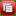Print AllEmail NotificationRSSSearch this Product Main Features Executive Summary Implementation Plan Calculating Contributions to Percentage Change (Appendix) About this Release APPENDIX CALCULATING CONTRIBUTIONS TO PERCENTAGE CHANGE INTRODUCTION 1 As of the December quarter 2017, the Australian Bureau of Statistics (ABS) will move to annual re-weighting of the Consumer Price Index (CPI) expenditure class (EC) weights. The Household Expenditure Survey (HES) will continue to be used to update the weights in the years that it is available. For the inter-HES years, Household Final Consumption Expenditure (HFCE) data from the National Accounts will be used to re-weight the CPI ECs. 2 These short-term CPI series will be chain-linked together to form longer term series. In consultations with users about this change, a number of stakeholders sought advice from the ABS to document methods to calculate contributions to percentage change with annually re-weighted indexes. 3 This appendix explains how the contributions of ECs to quarterly and annual CPI percentage changes are currently derived, then outlines how these contributions can be calculated under an annually re-weighted CPI. An approximation is provided for contributions to the annual inflation rate with an annually re-weighted CPI, which distinguishes between the effect of price changes of the ECs, and changes in the expenditure shares(footnote 1) . 4 The ABS will conduct empirical testing of the method outlined and publish the results in an information paper, which will also introduce the new CPI weights, to be released in November 2017. CURRENT PRACTICE 5 The current Australian CPI is a Lowe-type price index. This is an index where at the upper level of aggregation, the price indexes of the ECs are aggregated using fixed quantity, price-updated weights. 6 Points contributions allow users to understand how much an EC contributes to the overall All groups CPI. Points contributions for each EC are published in Tables 7 and 12 of the Time Series Spreadsheets released with the CPI each quarter (cat. no. 6401.0). The weight of EC n to the All groups CPI in quarter t can be estimated using points contributions:(1) where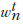is the weight (%) of EC n to All groups CPI,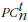is the points contribution of EC n and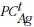is the points contribution (or price index) of the All groups CPI, all in quarter t. 7 These weights can be used in the calculation of contributions to the percentage change(footnote 2) . Under a Lowe-type price index, the contribution of individual EC n to the quarterly percentage change in the All groups CPI in quarter t is given by: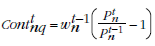(2) where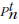represents the price index of EC n in quarter t. Note that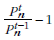is the percentage change of EC n between quarter t-1 and t. 8 The sum of the quarterly contributions of all ECs from (2) is equal to the quarterly percentage change in the All groups CPI. 9 Similarly, the contribution of EC n to the annual percentage change in the All groups CPI is given by the following: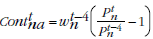(3) where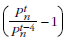is the annual percentage change of EC n. ANNUAL RE-WEIGHTING AND CHAINING 10 Under annual re-weighting, short-term CPI series (five quarters in length) will be constructed, each with their own set of weights. These series will then be chain-linked together to form a longer continuous time series. For an explanation and examples of chaining, see paragraphs 12.9 to 12.13 of the CPI Concepts, Sources and Methods publication (cat. no. 6461.0). 11 Under annual re-weighting, each quarterly percentage change of the chained CPI utilises a single set of weights. Therefore, calculating contributions to quarterly percentage change of the chained CPI does not pose an issue and can be derived in the same way as is currently done. For example, the contribution of EC n to the All groups CPI quarterly percentage movement in December quarter 2018 would be given by the following: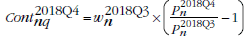(4) where 2018Q3 and 2018Q4 refer to the third and fourth quarters of 2018 respectively (i.e. the September and December quarters). This is the same expression as (2). Again, summing these contributions for all ECs will equal the quarterly percentage change in the All groups CPI in the December quarter 2018. DECOMPOSING ANNUAL PERCENTAGE CHANGES 12 Annual re-weighting does however pose a challenge when calculating contributions to the annual percentage change in the CPI. This is because the contribution will depend not only on changes in prices, but also changes in the weights. 13 As chaining is a multiplicative operation, and due to the arithmetic form of the short-term price index, it is not possible to calculate exact contributions of the ECs to the annual percentage change of the chained CPI. However, an approximation can be derived which distinguishes between the effects of annual percentage price change, and changes in the weights of the ECs. For the contribution of EC n to the annual percentage change between March quarter 2018 and March quarter 2019, the approximation is given by: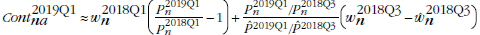(5) where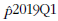is the price index for the All groups CPI in March quarter 2019 using the weights of the previous short-term series and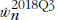is the weight of EC n in September quarter 2018 under the previous short-term series. 14 The first term in the expression represents the contribution due to the annual percentage price change, while the second term measures the contribution due to relative quantity (or weight) changes. The latter term is calculated with reference to the September quarter, which is the link quarter that connects the short-term series. 15 The ABS will provide additional information on the derivation of the approximation to interested users upon request. Requests can be sent to prices.statistics@abs.gov.au. 16 Further work will see the ABS conduct empirical testing of the decomposition shown in (5). The results of this will be published in an information paper to be released in November 2017. CONCLUSION 17 As of the December quarter 2017, the ABS will move to annually updating the CPI EC weights. The primary data source to update the weights for the inter-HES years will be National Accounts HFCE data. 18 Updating the EC weights and chain-linking the short-term (Lowe-type) CPI series in this way does not change the method of calculating EC contributions to quarterly percentage changes. This is because each quarterly percentage change of the chained CPI utilises a single set of weights. The contributions are calculated in the same way as has traditionally been done for the Lowe-type CPI with fixed quantity weights. 19 However, with annually updated weights, the contribution of an EC to the annual percentage change in the All groups CPI has to be approximated. A decomposition has been provided in this appendix which distinguishes between the contribution due to price change, and that due to changes in the weights. The ABS will provide additional information on the derivation of the approximation to interested users upon request. 20 The ABS will conduct empirical testing of the decomposition and publish the results in a November 2017 information paper. 1 The ABS acknowledges the input and advice provided by Professor Jan de Haan of Statistics Netherlands and Delft University of Technology in the development of this method.